# 背景介绍

``````# http://www.scikit-yb.org/zh/latest/quickstart.html
# http://www.scikit-yb.org/en/latest/quickstart.html
``````
``````import pandas as pd
X = data[[
"season", "month", "hour", "holiday", "weekday", "workingday",
"weather", "temp", "feelslike", "humidity", "windspeed"
]]
y = data["riders"]
``````
``````from yellowbrick.features import Rank2D
visualizer = Rank2D(algorithm="pearson")
visualizer.fit_transform(X.values)
visualizer.poof() # 在notebook显示
# visualizer.poof(outpath="pcoords.jpg",clear_figure=True)  # 输出为png、jpg格式
``````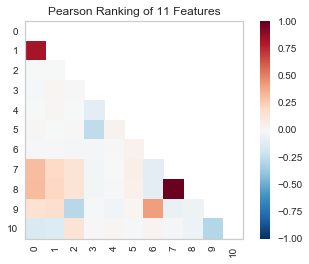``````from yellowbrick.features import JointPlotVisualizer
visualizer = JointPlotVisualizer(feature='temp', target='feelslike')
visualizer.fit(X['temp'], X['feelslike'])
visualizer.poof()
``````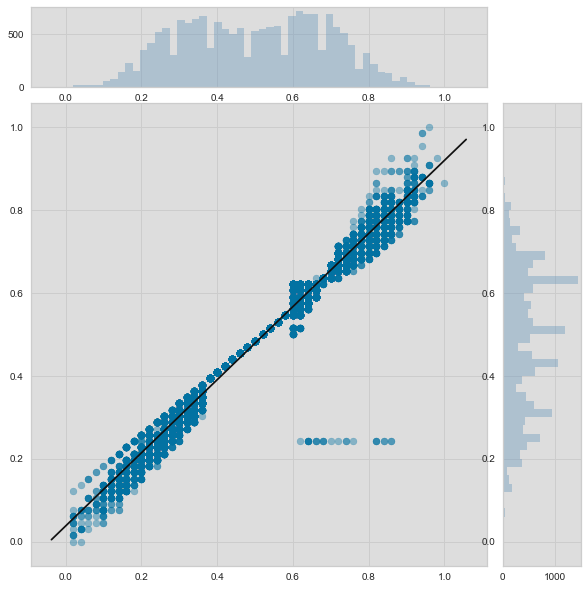JointPlotVisualizer 让我们能快速浏览有强相关性的特征，以及各个特征的范围和分布情况。需要注意的是图中的各个轴都已经标准化到0到1之间的值，这是机器学习中一中非常常用的减少一个特征对另一个影响的技术。

``````from yellowbrick.regressor import ResidualsPlot
from sklearn.linear_model import LinearRegression
from sklearn.model_selection import train_test_split

# Create training and test sets
X_train, X_test, y_train, y_test = train_test_split(
X, y, test_size=0.1
)

visualizer = ResidualsPlot(LinearRegression())
visualizer.fit(X_train, y_train)
visualizer.score(X_test, y_test)
visualizer.poof()
``````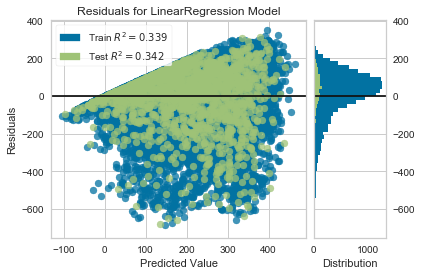``````import numpy as np

from sklearn.linear_model import RidgeCV
from yellowbrick.regressor import AlphaSelection
# RidgeCV:多个阿尔法，得出多个对应最佳的w,然后得到最佳的w及对应的阿尔法
alphas = np.logspace(-10, 1, 200)
visualizer = AlphaSelection(RidgeCV(alphas=alphas))
visualizer.fit(X, y)
visualizer.poof()
``````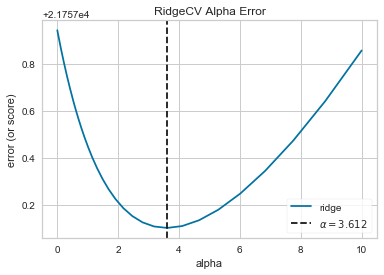``````alpha = visualizer.alpha_
visualizer.alpha_
``````
``````3.612342699709438
``````

``````from sklearn.linear_model import Ridge
from yellowbrick.regressor import PredictionError

visualizer = PredictionError(Ridge(alpha=alpha))
visualizer.fit(X_train, y_train)
visualizer.score(X_test, y_test)
visualizer.poof()
``````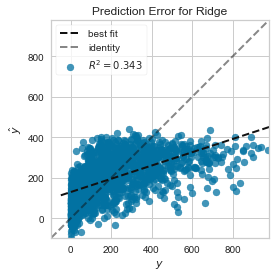``````
``````

05/05
0
0

Python是目前学习数据分析课程中，非常重要的一块，也是目前非常受行业欢迎的。因此，学习非常重要，下面是Python学习的一些重要步骤和知识！ 步骤1：熟悉Python 对数据科学，Python和R都是不...

weixin_41852491
2018/05/28
0
0
Python 机器学习的必备技巧

2018/11/08
0
0

Yellowbrick是一套视觉诊断工具，它扩展了Scikit-Learn API，允许人为操纵模型选择过程。 简而言之，Yellowbrick将scikit-learn与matplotlib结合在scikit-learn文档的最佳实践中，但为您的模...

08/21
448
0
PyCon2018：两款最新ML数据可视化库：Altair和Yellowbrick

Altair例程
2018/12/06
0
0

Centos7 python2.7和yum完全卸载及重装

完全重装python和yum 1 2 3 4 5 6 7 8 9 10 11 12 13 14 15 16 17 18 19 20 21 22 23 24 25 26 27 1、删除现有...

roockee
25分钟前
5
0
【软件工程】绪论，深入浅出理解软件工程

32分钟前
5
0

7
0

6
0
Kubernetes 从懵圈到熟练：集群服务的三个要点和一种实现

11
0